## Tricolor automata# Proceedings

## Tricolor automata

Balisage: The Markup Conference 2015
August 11 - 14, 2015

## The problem

### Background

Complex public vocabularies sometimes come in multiple flavors (such as the Transitional and Strict variants of HTML 4.0, or the Archiving, Publishing, and Authoring variants of the Journal Archiving Tag Suite [JATS]); any vocabulary that is used long enough is likely to come in multiple versions (as do HTML, JATS, DocBook, TEI, and others). Since many public vocabularies are designed to be customizable for a given use (and any vocabulary at all may be customized, whether designed for it or not), they will also come in customized and non-customized forms.

Users (and maintainers) of such vocabularies will from time to time wish to understand just how two variants of a vocabulary resemble each other and how they differ from each other. Those preparing a new version of a vocabulary may wish to verify that all documents valid against the old version remain valid, or to see concisely exactly which have been invalidated; those working with a customized vocabulary may wish to understand better just how it differs from the base vocabulary. And so on. In practice, such understanding seems to require the pairwise comparison of corresponding declarations in the two schemas.

We can of course compare the two declarations textually. This sometimes turns out to be less helpful than one might wish. Here is the declaration of the `body` element in one customization of the TEI (reformatted for legibility):

```<!ELEMENT body ((%model.global;)*,
((%model.divTop;),
(%model.global;|%model.divTop;)*)?,
(_DUMMY_model.divGenLike,
(%model.global;
|_DUMMY_model.divGenLike)*)?,
(((%model.divLike;),
(%model.global;
|_DUMMY_model.divGenLike)*)+
|(_DUMMY_model.div1Like,
(%model.global;
|_DUMMY_model.divGenLike)*)+
|(((%model.common;),
(%model.global;)*)+,
(((%model.divLike;),
(%model.global;
|_DUMMY_model.divGenLike)*)+
|(_DUMMY_model.div1Like,
(%model.global;
|_DUMMY_model.divGenLike)*)+)?)),
((%model.divBottom;),
(%model.global;)*)*)>```

And here is another:

```<!ELEMENT body ( (%model.global;)*,
( (%model.divTop;),
(%model.global; | %model.divTop;)*
)?,
( ( (%model.divLike;),
(%model.global;)* )+
| ( ( (%model.common;),
(%model.global;)* )+,
( ( (%model.divLike;),
(%model.global;)* )+ )? )
),
( (%model.divBottom;),
(%model.global;)*
)*
) >```

So many details are hidden behind the parameter entities (or in a Relax NG schema behind the named patterns, or in an XSD schema behind the named types, type inheritance, and named model groups) that it is difficult to see, from the textual form of the declaration, just where the two content models are the same and where they differ. If we expand the content models, with content models this complex, the situation does not become better.

Drawing a finite-state automaton (FSA) of a content model can sometimes make the structure clearer (although in the case of complex models like these, there are so many states in the automata that careful study may be needed). Here is the first declaration, in FSA form:

Figure 1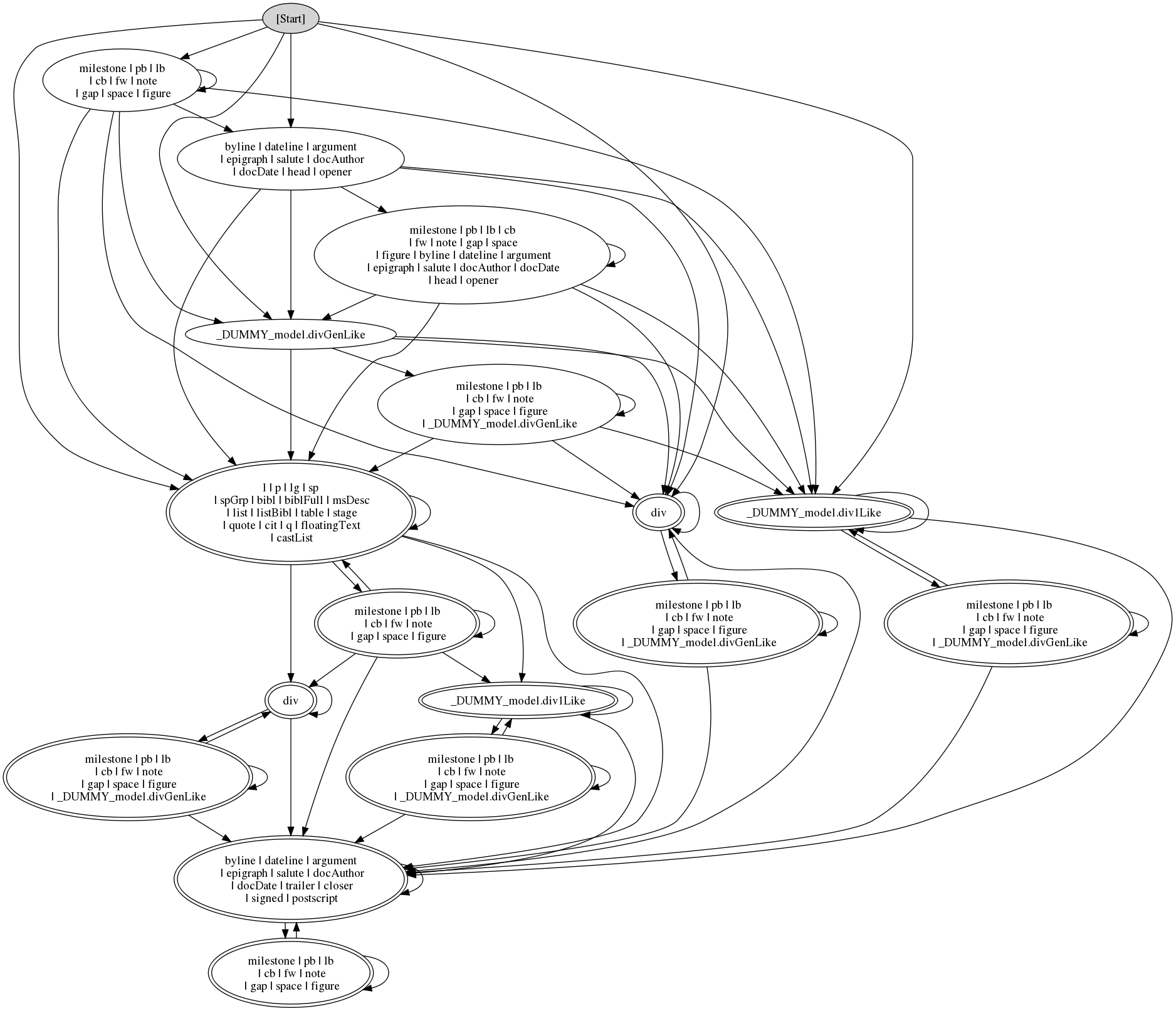Here is the FSA for the second declaration:

Figure 2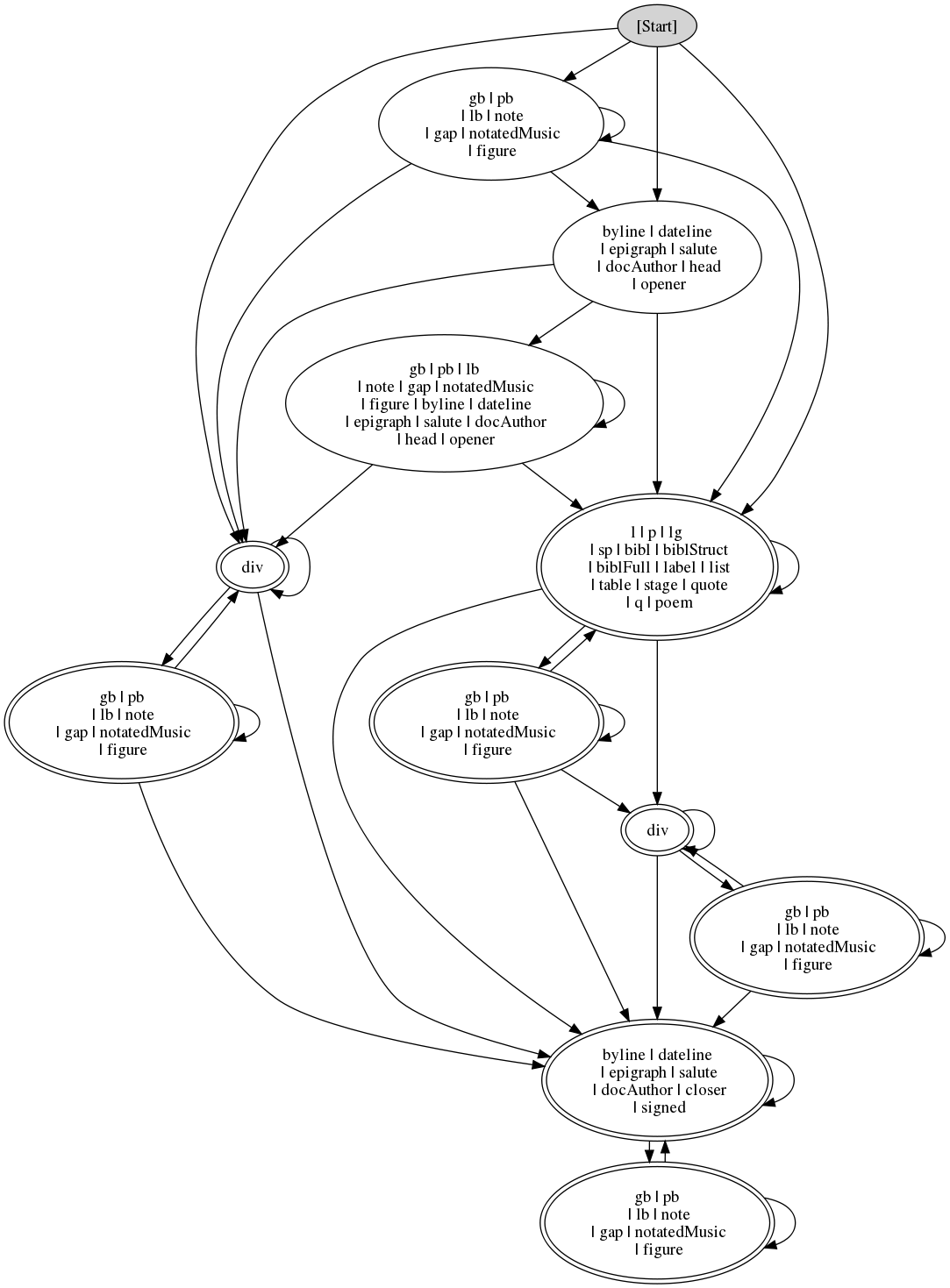The FSAs may be easier to understand, in some ways, than the textual declarations, but comparing them does not seem materially easier. Can we do better?

### Desiderata

Informally, the problem is: given two regular expressions A and B, draw a circle-and-arrow diagram which allows the user to see as clearly as possible what the FSAs look like for languages L(A), L(B), L(A∩B), and L(A∪B).

This is informal because as clearly as possible implies some sort of optimization, and we don't have a clear notion of optimality. Intuitively,

1. Fewer states is better than more states. (Ditto arcs.)

2. Determinism is better than non-determinism.

3. Having the Glushkov property (see below) is better than not having it.

4. Having a relatively clear and simple relation between the circles of the diagram and (preferably contiguous) portions of the regular expression (or content model) is better than having a more complex relation.

We know from automata theory that these desiderata may conflict: Glushkov automata are often not minimal, and determinizing an NFSA sometimes adds states. In the general case, then, there may be multiple forms of optimality based on different priorities among these three desiderata.

### The Glushkov Property

The term Glushkov property is not standard, so it should be explained. The Russian mathematician V.M. Glushkov described an algorithm for making an FSA from any regular expression, with n+1 states, where the regular expression contains n letters [Glushkov 1961]. (Or in SGML/XML terms, where the content model contains n primitive content tokens — `#PCDATA` or a generic identifier.) Anne Brüggemann-Klein and D. Wood pointed out that the ambiguity rule of SGML amounted to this: the Glushkov Automaton built from a content model must be deterministic [Brüggemann-Klein 1993a], [Brüggemann-Klein 1993b], [Brüggemann-Klein/Wood 1998]. If it's not, there is what SGML describes as an ambiguity error.

Now, since in a Glushkov automaton we have one state per letter in the regex, we can simply say the states of the Glushkov automaton are the letters (i.e. the letter tokens) in the regex. So each state is a token in the regex. And every incoming transition to a state q is labeled with the type of q. So the content model `(a, (b|c)*, d)` has five states: one start state and one state for each of the tokens `a`, `b`, `c`, and `d`. There is a transition from `a` to `b`, labeled `b`, and a transition from `a` to `c`, labeled `c`. And (because the choice of `b` and `c` has an asterisk as its occurrence indicator), there is also a transition from `a` to `d`, labeled `d`. There are also transitions from `b` and `c` to `b` and `c`, labeled `b` and `c`. And from the same two states there is a transition to `d`, labeled `d`.

Figure 3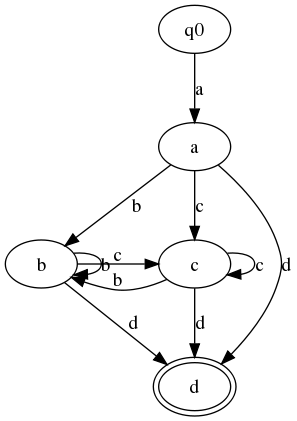Note that every incoming transition to `b` carries the same label, namely `b`, and similarly for nodes `c` and `d`.

Since in a Glushkov automaton every incoming transition to a state q is labeled with the type of q, it follows that when we draw Glushkov automata, we can label the states and leave the transitions unlabeled.

Figure 4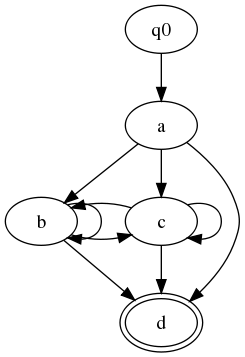We know what label a transition has, because we can read it from the target state. Some readers may find that Glushkov automata remind them a bit of Moore machines. In each cases each state in the automaton is associated with a single symbol: an output symbol, in the case of a Moore machine, and an input symbol, in the case of the Glushkov automaton.

In general, however, FSA edges may be labeled with more than one symbol. A minimal FSA for the expression `(a, (b|c)*, d)` will have only three states, not five:

• q0 (the start state)

• q1 (reached after any `a`, `b`, or `c` in matching input)

• q2 (the final state, reached after `d` in matching input)

The FSA will have the following arcs:
• q0q1 on `a`

• q1q1 on `b` or `c`

• q1q2 on `d`

Figure 5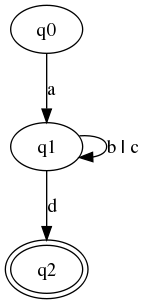The cycle on state q1 is labeled `b | c`; the alternative is to have two arcs, one for each label, but the figure is less cluttered if there is only one arc between any two states; that arc may be labeled with a set of symbols, not a single symbol.

We say that such an FSA has the Glushkov property if for any state q, all of q's incoming arcs have the same set of labels. Thus (just as for a Glushkov automaton), we can label each state q with the set of symbols that can reach q from some other state (more formally: the set of symbols which can appear as the last symbol in a sequence that starts at the start state and ends at q).

Note that all Glushkov automata have the Glushkov property, but not all automata with the Glushkov property are Glushkov automata. The two FSAs for customizations of the TEI `body` element shown above, for example, have the Glushkov property, but each or-group in the content model is represented by a single state, rather than one state for each primitive content token in the or-group. This dramatically reduces the size and complexity of the FSA for some content models, and makes the FSA more legible. Just as with any FSA, if any two equivalent states in a Glushkov automaton are merged, the resulting FSA will recognize the same language as the original. To retain the Glushkov property, however, we must impose the additional constraint that the two states must not only be equivalent (both in or both outside the set of final states, and both with transitions to the same set of following states), but also have incoming transitions from the same set of preceding states.

The reason we want the Glushkov property is that it makes the drawings easier to draw and (so it seems) easier to understand. This may be related to the apparently common view that Moore machines are easier to understand than Mealy machines, even though they may have more states.

## Tricolor automata

Tricolor automata are an attempt to solve the problem outlined above. The basic idea is to draw an FSA (or more precisely: a circles-and-arrows diagram), in which each state and each transition arc is colored: white states and black arcs are reachable or traversible in both languages (i.e. in L(A∩B)), red states and arcs belong to L(A) but not to L(B), and blue states belong to L(B) but not to L(A).

If when generating or walking through a string, or the content of an element, we stick to the white states and end in a state drawn with a black double circle, then the string or element is in both L(A) and L(B). If the path we trace touches only white states and red states, including at least one red state or red arc, and ends in a state with a black or a red double circle, then the string or element is in L(A) but not in L(B). And if the path we trace touches only white states and blue states, including at least one blue state or arc, and ends in a state with a black or a blue double circle, then the string or element is in L(B) but not in L(A). (And of course if the string or element doesn't match any path through the FSA, then it is in neither language.)

There are at least two ways to draw diagrams with these properties, which I call the tainted-string and color filter approaches.

### The tainted-string approach

The tainted-string approach draws an FSA in which we can traverse from white states to red or blue states, but not vice versa: once we are in a red state we are always thereafter in red states, and similarly for blue states. (This might be called the McCarthy principle: once a red, always a red.)

For example, let A = `(a|x)*, z`:

Figure 6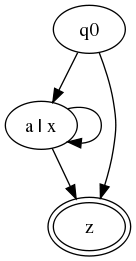Let B = `(b|x)+, z`.

Figure 7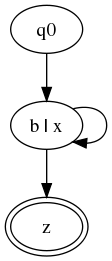A simple algorithm for translating from content models to tricolor automata will produce something like the following:

Figure 8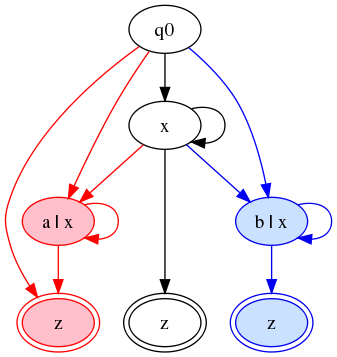There are two problems with this, however: First, in state q0, symbol `x` is non-deterministic: it can go to any of three places. If we want blue and red to mean B-only and A-only, we don't want `x` going to red or blue states. One way to fix this would be to label the arcs and not the states:

Figure 9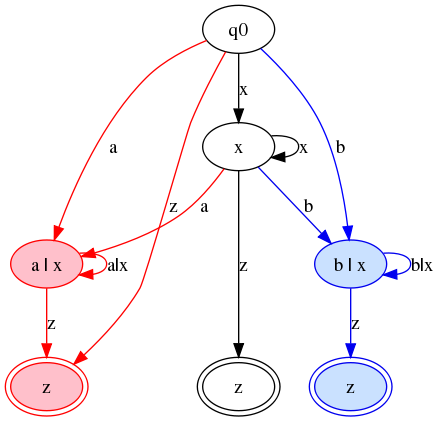It is perhaps only a subjective view, but speaking for myself I find that harder to read, and in more complicated cases — as illustrated by almost any content model from a real public vocabulary — it's even worse.

And the automaton has now lost the Glushkov property: the node labeled a|x has two incoming arcs labeled a and a third incoming arc labeled a|x; the node labeled b|x has similarly inconsistent labels on incoming arcs. We can regain the Glushkov property and get back to labeling states by adding new states:

Figure 10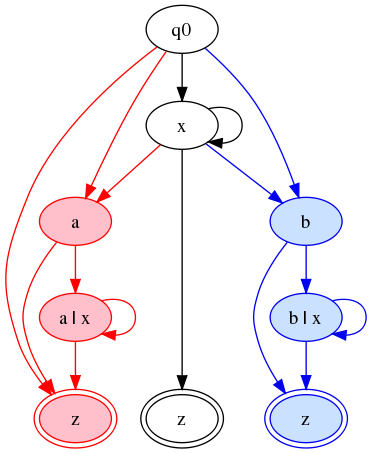This is a reasonably clear graph. It's deterministic, and it has the Glushkov property, and it is the smallest FSA I have found that has those properties. Its treatment of the three final states illustrates very nicely the tainted-string approach: we cannot use a single state for `z`, because the taint approach says that from a red state, only red states are reachable.

### The color-filter approach

If we relax the McCarthy principle and allow transitions from red or blue states to white states, in order to reduce the size of the automaton, the first cut looks like this:

Figure 11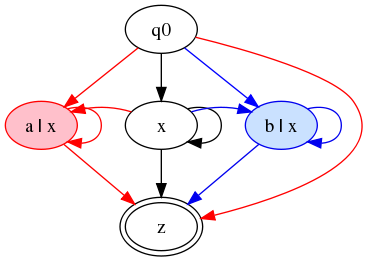However, this is again non-deterministic. What I think we want is something like this:

Figure 12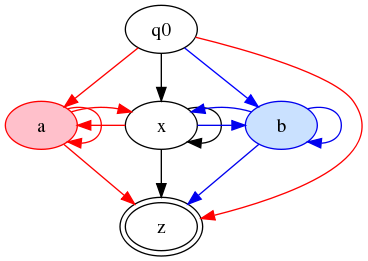Note that this example shows that in the color-filter approach, coloring the arcs is necessary, because q0 → z is only legal in L(A)); in the tainted-string approach, arcs need not be colored.

### A complication

Consider the two expressions A = `(a|x)*, z` and B = `(b|x)*, z?`. (That is, A is as above, and B is modified by making the closing `z` optional.)

The diagrams we want will be very similar to the final versions of the tainted-string and color-filter diagrams shown above, but they must change to reflect the fact that the states `b`, `x`, and `b | x` are all now final states in expression B.

This forces us into a choice. In the examples given above, both of the following rules hold true:

• Any state has one color, and is either a final state or not. The final or non-final status of the state is independent of its color.

• It for each tricolor automaton, we have been able to construct an equivalent tricolor automaton which is deterministic and has the Glushkov property.

This example seems to allow us to retain at most one of these generalizations.

If we retain the first and abandon the second, we will end up with a diagram like this for the tainted-string approach:

Figure 13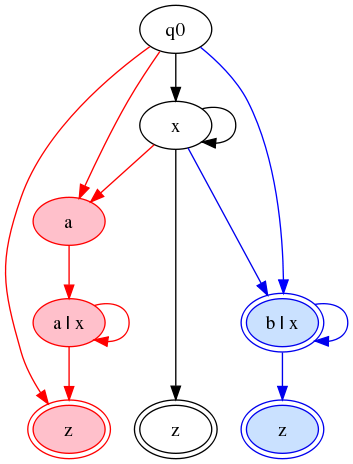For the color-filter approach, we get:

Figure 14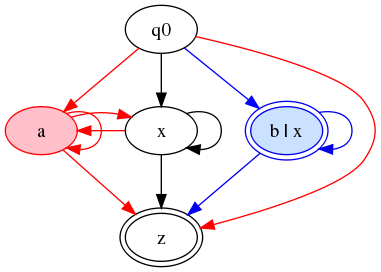Note that in both cases, we have lost determinism: an initial `x` goes either to the white state `x` or the blue final state `b | x`.

We can retain determinism if we adopt the convention that a white state can be marked final either in black (as above), or in red or blue. In the latter case, it means that the state is a final state for L(A) but not L(B), or vice versa.

On this principle, the tainted-string approach gives us a diagram like this:

Figure 15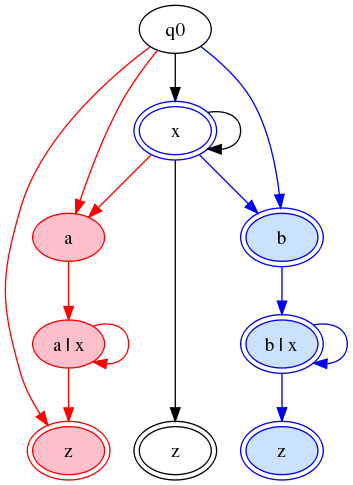And the color-filter approach produces:

Figure 16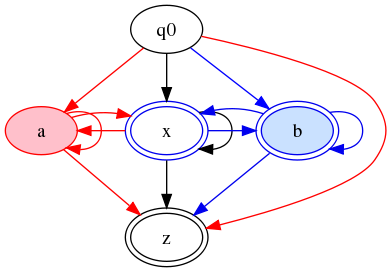At the beginning of this section, I said we must choose between determinism and the rule that finality of a state is independent of color. Actually, we do not have a real choice, as should be clear from consideration of the pair A = `(a|x)*, z?` and B = `(b|x)*, z`. Here the initial state is final in A, but not in B. Unless we want to assume multiple possible initial states (and rewrite as much of standard automata theory as necessary in order to deal with the change), we must have a way to indicate that a state may be visited by strings in either L(A) or L(B), but is final in only one of those languages.

### Interpreting the diagrams

So, what does this circle-and-arrow diagram mean? I believe it describes, concisely, how to construct strings in:

• L(A): visit only red or white states along red or black arcs; end in a state marked final in red or black.

• L(B): visit blue or white states along blue or black arcs; end in a state marked final in blue or black.

• L(A∩B): visit only white states, using only black arcs; end in a state marked final in black.

• L(A∪B): use no more than two colors of state or arc (red + white or blue + white — we cannot construct any just-red-and-blue paths because the start state is black/white). End in a state marked final in one of the two colors.

• L(A) \ L(B): use only white and red states; use at least one red arc; end in a state marked final in red or black.

• L(B) \ L(A): use only white and blue states; use at least one blue arc; end in a state marked final in blue or black.

Note that if we ignore colors entirely, the circles-and-arrows diagram describes an FSA for an expression something like ```(a | b | x)*, z```. This is not the union of L(A) and L(B) (it's larger), but it is close to what some document designers (including me) would probably write, if someone asked for a grammar that would accept both A documents and B documents and also would look like a rational document grammar. In some real-world cases, effective content models like `((a|x)*, z)` and `((b|x)+, z)` arise as expansions of `((%some-element-class;)*, z)` and `((%some-element-class;)+, z)`: the two effective models differ because (a) the two versions have customized the some-element-class class differently and (b) vocabulary B has required at least one occurrence of an element in the class (or, possibly, A has made it optional). The natural union schema for A and B in this case would define the class some-element-class to include `a`, `b`, and `x` (the union of its members in vocabularies A and B), and define the content model as `((%some-element-class;)*, z)`, which would expand to `(a | b | x)*, z`.

The one catch is that this is not, strictly speaking, an FSA. The characteristic of an FSA is that the only memory it has is the identity of its current state. When a string ends, we are allowed to ask Is the current state a member of the set of final states? and that's it. Keeping track of what colors we have seen along the way requires memory that goes beyond keeping track of the current state.

It's clear, however, that from such a graph we can readily construct an FSA for the first four of the languages identified above, by dropping out the states and arcs that are outlawed in the rule for that language. For the last two languages listed, it may not be straightforward to construct a free-standing FSA; that it is possible is a straightforward fact of automata theory.

### Formal definition

We are now in a position to define a tricolor automaton more formally.

A tricolor automaton is a triple (A, Χ, Φ), where

• A is the underlying finite state automaton (Q, Σ, δ, q0, F), where

• Q is a set of states

• Σ is an input alphabet

• δ is a transition function mapping from Q × Σ to Q

• q0Q is the start state

• FQ is the set of final states

• Χ is a chromatic function mapping from Q ∪ δ to the colors {red, white, blue}.

• Φ is a chromatic function mapping from F to the colors {red, white, blue}.

Given the intended uses of tricolor automata as outlined in the preceding section, some possible chromatic functions will make no sense in practical applications. Arcs connecting red and blue states, red arcs incident to blue states, blue arcs incident to red states, white arcs connecting pairs of red or pairs of blue states, can play no role in the strings of any of the languages of particular interest. Similarly if a red state is mapped by Φ to any color but red, the conventional implication is that the state is final in B, but the state cannot be reached by any string in L(B), so the mapping in Φ has no effect on any language except that of the base FSA. (And analogously for any blue states mapped by Φ to any color but blue.)

In practical applications, therefore, the chromatic functions Χ and Φ will normally obey the consistency constraints that

• For any two states q1 and q2 connected by an arc a from q1 to q2:

• {Χ(q1), Χ(q2)} ≠ {red, blue} (no arcs connect red and blue states directly).

• If red ∈ {Χ(q1), Χ(q2)}, then Χ(a) = red (any arc incident to a red state is red).

• If blue ∈ {Χ(q1), Χ(q2)}, then Χ(a) = blue (any arc incident to a blue state is blue).

• If Χ(q) = red, then Φ(q) ∈ {red}.

• If Χ(q) = white, then Φ(q) ∈ {red, white, blue}.

• If Χ(q) = blue, then Φ(q) ∈ {blue}.

## How to create tricolor automata

The simplest way to create a tricolor automaton is to extend the rules for creating an FSA from a regular expression using Brzozowski derivatives [Brzozowski 1964].

As some readers will recall, given a regular expression E, the derivative of E with respect to some symbol (or string) s is an expression E′ such that L(E′) is the set of strings t such that concat(s,t) is in L(E). Informally, E′ is an expression that records what we still expect to see in the string, after s, if the string as a whole is going to match the original expression E. Note that the derivative E′ may be , meaning that there is no string t which can be concatenated with s to form a string in L(E)

Brzozowski's method of building an FSA is: start with the original expression E, and take the derivative of E with respect to each symbol in the alphabet. Simplify and normalize those expressions. Remove duplicates, and remove any that match any expression already seen (like E itself). Continue until you have taken the derivative of F with respect to s for each expression F in the set of expressions seen so far and each symbol s in the alphabet. Brzozowski proves that this process terminates, even if the process of detecting and removing duplicates is slightly imperfect; he provides a set of simple equivalences which are not exhaustive but suffice to guarantee termination.

Now, the FSA is built this way: Each expression in the resulting set of expressions becomes a state in the FSA. The original expression E is the start state. An expression is a final state if and only if it accepts the empty string. And for any two states F and G, the transition function includes the transition FG on some symbol s if and only if the derivative of F with respect to s is G.

We modify this algorithm in two ways, so as to ensure that result is a tricolor automaton and has the Glushkov property.

First: instead of starting out with a single expression E, we start with the two expressions A, B, A and B, A or B. Accordingly, instead of a set of regular expressions, what we generate is a set of pairs (DA, DB), where DA is an expression derived ultimately from A, DB from B. Working with pairs of expressions allows us to associate the appropriate color with each state and arc: states in which both DA and DB define some non-empty language (accept at least one sequence of input) are white; states in which DA is non-empty and DB is {} (the empty set) are colored red, and states in which DA is {} and DB is non-empty are colored blue. (States of the form ({}, {}) are error states and are omitted for compactness of the resulting FSA.)

Second: instead of making a state for each distinct pair of expressions we encounter, we distinguish states based on a triple (s, DA, DB), where s is a set of symbols in the input alphabet, and DA and DB are as described above. It's easiest to follow examples (and implement) if we assume the symbol sets are all singletons, but in practice we want to take the largest sets we can in order to have fewer states in the result, so after generating an initial tricolor automaton, we will merge states which can be safely merged.

### The tainted-string approach

The simplest case uses the tainted-string approach. Here, we identify states based on the strict identity of the triple. We can describe the algorithm as follows. We keep a list of triples seen so far, and mark each triple when it is processed.

In what follows, it will be convenient to define the derivative of a triple (s1, DA, DB) with respect to some input symbol s as the triple (s, DA′, DB′), where DA′ is the result of simplifying the derivative of E with respect to s and DB′ is the result of simplifying the derivative of DB with respect to s.

1. Initially, the triple list contains the single triple ('', A, B), where A and B are the expressions for which we are creating the tricolor automaton.

2. Consider the first unprocessed triple in the list. Call it T; calls its two expressions TDA and TDB.

3. For each symbol s in the input alphabet:

1. Calculate T′, the derivative of T with respect to s; it will take the form (s,TDA′, TDB′).

2. If the triple (s, TDA′, TDB′) is already in the triple list, do nothing.

3. Otherwise add it to the end of the triple list.

4. If there are any unprocessed triples in the list, return to step 2.

5. Otherwise, stop.

The process is guaranteed to terminate, because the number of non-equivalent DA and DB which can be generated from A and B is finite.

The resulting tricolor automaton and its underlying finite state automaton A can be described thus:

• The states Q of A are the entries in the triple list upon termination of the process.

• The alphabet Σ of A is the union of the alphabets of A and B.

• For any states q1 and q2 and any symbol s, the transition function δ of A has an arc from q1 to q2 if and only if q2 is the derivative of q1 with respect to s.

• The initial state q0 of A is the triple ('', A, B).

• The final states F of A are those triples (s, DA, DB) for which either DA or DB is nullable (accepts the empty string).

• The chromatic function Χ maps states q = (s, DA, DB) to colors in this way:

• If DA and DB = , then Χ(q) = red.

• If DA = and DB, then Χ(q) = blue.

• If DA and DB, then Χ(q) = white.

Χ maps all arcs that begin or end in a red state to red, all arcs that begin or end in a blue state to blue, all others to white (which, perhaps confusingly, is here drawn in black).

• The chromatic function Φ maps final states q = (s, DA, DB) to colors in this way:

• If Χ(q) ∈ {red, blue}, then Φ(q) = Χ(q).

• If Χ(q) = white, then:

• If DA accepts the empty string, and DB does not, then Φ(q) = red.

• If DB accepts the empty string, and DA does not, then Φ(q) = blue.

• If both DA and DB accept the empty string then Φ(q) = white.

Note that the case where neither DA nor DB accepts the empty string cannot arise, because such states are not in the set F of final states.

The logic of the coloring scheme is this. Any state in which DA = ∅ is outside the language of A, so it's B-only, and any state with DB = ∅ is outside the language of B, so it's A-only. We color them blue and red, respectively. Any state with neither DA nor DB = ∅ is at least potentially in the intersection, so it's white. And any state with DA = DB = ∅ is of no interest so we drop it.

Since any state with DA ≠ ∅ is colored white or red, any path restricted to white and red states and arcs

A similar technique can be used to create a tricolor automaton from two FSAs instead of two regular expressions. It resembles the usual algorithm for calculating an FSA for the intersection of two languages recognized by FSAs, but its states are again triples, which consist of an input symbol, a state from the first FSA (or a flag indicating that there is no applicable state), and a state from the second FSA (or a not-applicable flag).

### An example

The algorithm can be illustrated by applying it to the example used above.

• A = (a|x)*, z

• B = (b|x)+, z

• Σ = {a, b, x, z}

At the outset, our list of triples has just one entry, for the start state:

• q0 (start state) = ('', A, B)

For the two expressions A and B, the derivatives with respect to the symbols of the alphabet are as follows:

• deriv(A,`a`) = A

• deriv(A,`b`) = ∅

• deriv(A,`x`) = A

• deriv(A,`z`) = ''

• deriv(B,`a`) = ∅

• deriv(B,`b`) = `(b|x)*, z`

• deriv(B,`x`) = `(b|x)*, z`

• deriv(B,`z`) = ∅

Let's give the name B′ to the expression `(b|x)*,z`, for brevity. From these, we can calculate the derivatives of triple q0:
• deriv(q0,a) = (a, A, ∅)

• deriv(q0,b) = (b, ∅, B′)

• deriv(q0,x) = (a, A,B′)

• deriv(q0,z) = (z, '', ∅)

Each of these is new and is added to the list:
• q1 = (a, A, ∅)

• q2 = (b, ∅, B′)

• q3 = (a, A, B′)

• q4 = (z, '', ∅)

The fragment of the automaton thus far constructed is this:

Figure 17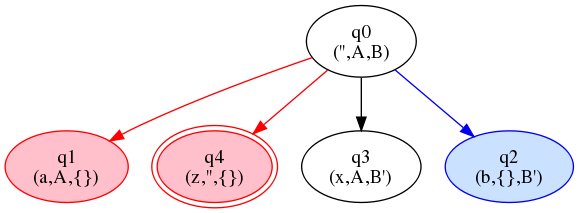We now take the derivatives of each of these new states:

• q1:

• deriv(q1,a) = q1

• deriv(q1,b) = (b, ∅, ∅)

• deriv(q1,x) = (x, A, ∅) becomes q5

• deriv(q1,z) = (z, '', ∅) = q4

• q2:

• deriv(q2,a) = (a, ∅, ∅)

• deriv(q2,b) = (b, ∅, B′) = q2

• deriv(q2,x) = (x, ∅, B′) becomes q6

• deriv(q2,z) = (z, ∅, '') becomes q7

• q3:

• deriv(q3,a) = (a, A, ∅) = q1

• deriv(q3,b) = (b, ∅, B′) = q2

• deriv(q3,x) = (x, A, B′) = q3

• deriv(q3,z) = (z, '', '') becomes q8

• q4: all derivatives are error states of the form (s, ∅, ∅).

At this point, we've added several new states (q5 through q8), and the graph looks like this:

Figure 18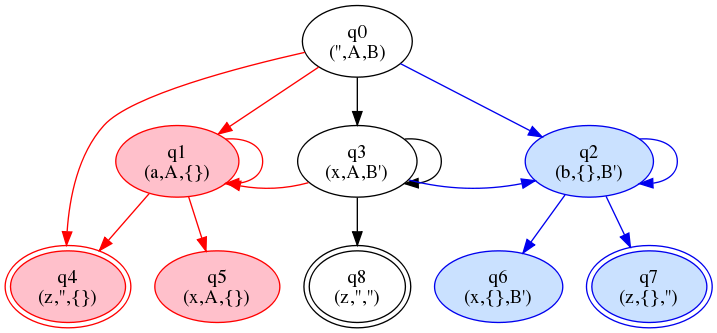Calculating derivatives for the new states produces no new states, but some new arcs:

• q5:

• deriv(q5,a) = q1

• deriv(q5,b) = (b, ∅, ∅)

• deriv(q5,x) = q5

• deriv(q5,z) = q4

• q6:

• deriv(q6,a) = (a, ∅, ∅)

• deriv(q6,b) = q2

• deriv(q6,x) = q6

• deriv(q6,z) = q7

• q7: deriv(q7,s) = (s, ∅, ∅) for all symbols s

• q8: deriv(q8,s) = (s, ∅, ∅) for all symbols s

The result of adding the new arcs is shown below.

Figure 19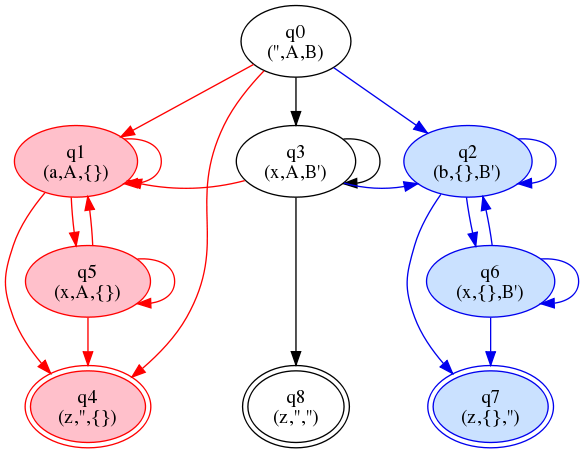Or, getting rid of the bookkeeping details:

Figure 20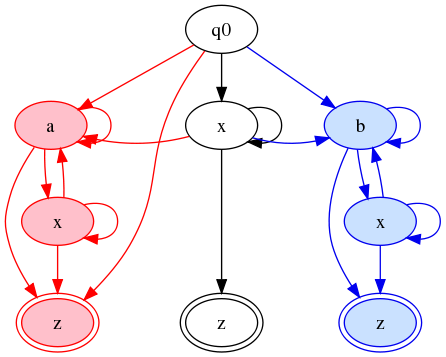This is almost but not quite the same as the last graph shown in the introductory section on the tainted-string approach above. By the desiderata mentioned at the outset, it is almost, but not quite, as good: it has the same number of states, but more arcs.

### The color-filters approach

This approach is just like the tainted-string approach, with just one difference: after construction of the automaton, we search for two states of the form (s, DA, DB) and (s DA, ∅) or (s, DA, DB) and (s ∅, DB). Each such pair we find is merged by keeping the first (full) form and dropping the other.

In our example, this leads to the merger of states q3 = (x, A, B′), q5 = (x, A, ∅), and q6 = (x, ∅, B′). It also leads to the merger of the three z-states q4, q8, and q7.

Figure 21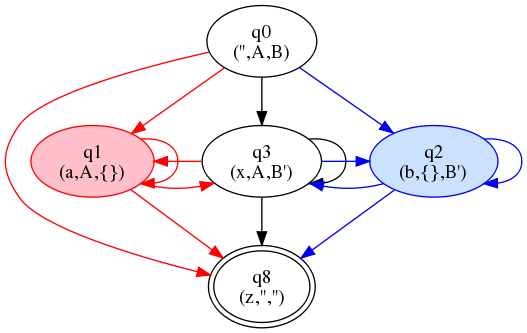Or, without 5-tuples:

Figure 22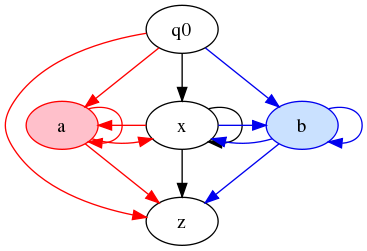This is isomorphic to the graph shown above in the introduction to the color-filter approach.

## Conclusion and further work

We have described (or discovered) the tricolor automaton, a form of multi-colored FSA. These differ in two ways from conventional FSAs: First, arcs and states may be colored in any of three colors (here red, black/white, and blue). Second, the final states of a tricolor automaton each map to one of the colors red, white, or blue (independently of the color of the state).

From any two expressions (or any two finite state automata) A and B, we can construct a tricolor automaton. The base FSA of the tricolor automaton defines a language which is a superset of the the union of L(A) and L(B), and can be used, together with the coloring of the states, arcs, and final sets, to identify several other languages: L(A) and L(B) themselves, L(A∩B), L(A∪B), L(A) \ L(B), and L(B) \ L(A).

By applying standard FSA minimization and determinization algorithms (with suitable modifications to ensure color correctness), we can make the graph relatively compact. We also have an algorithm for building the graph in such a way that it possesses the Glushkov property. (The simplicity with which Brzozowski derivatives can be used to create automata with the Glushkov property may be what Brüggemann-Klein and Wood have in mind when they say that there is an intimate relation between the Glushkov Automaton for an expression and its set of characteristic Brzozowski derivatives.)

Several avenues for further work appear likely to be fruitful. First, it would be helpful to have a more formal treatment, specifying the conditions under which the various desiderata (minimal states, minimal arcs, determinism, the Glushkov property, and a one-to-one or many-to-one relation between tokens in the content model or regular expression and states in the automaton) are compatible and when they are at odds with each other. When they cannot all be achieved, a clear formal description of the tradeoffs would be interesting. It would also be interesting and helpful to have a formal proof that the algorithms sketched here produce tricolor automata with the properties claimed.

An extension to arbitrary numbers of expressions and corresponding numbers of colors (n expressions and 2n - 1 colors) is obviously also possible and may be interesting (although for n greater than two, the number of colors involved probably makes the resulting diagrams useless for human inspection).

Finally, practical experience using tricolor automata in the comparison of actual models in published vocabularies will be necessary to determine whether they actually help address the problems identified in the introduction.

## References

[Brüggemann-Klein 1993a] Brüggemann-Klein, Anne. 1993. Regular expressions into finite automata. Theoretical Computer Science 120.2 (1993): 197-213. doi:https://doi.org/10.1007/BFb0023820.

[Brüggemann-Klein 1993b] Brüggemann-Klein, Anne. 1993. Formal models in document processing. Habilitationsschrift, Freiburg i.Br., 1993. 110 pp. Available at ftp://ftp.informatik.uni-freiburg.de/documents/papers/brueggem/habil.ps (Cover pages archival copy also at http://www.oasis-open.org/cover/bruggDissert-ps.gz).

[Brüggemann-Klein/Wood 1998] Brüggemann-Klein, Anne, and Derick Wood. 1998. One-unambiguous regular languages. Information and computation 140 (1998): 229-253. doi:https://doi.org/10.1006/inco.1997.2688.

[Brzozowski 1964] Brzozowski, Janusz A. Derivatives of regular expressions. Journal of the ACM 11.4 (1964): 481-494. doi:https://doi.org/10.1145/321239.321249.

[Glushkov 1961] Glushkov, V. N. 1961. The abstract theory of automata, tr. J. M. Jackson, in Russian Mathematical Surveys, A translation of the survey articles and of selected biographical articles in Uspekhi matematicheskikh nauk, ed. J. L. B. Cooper, vol. 16 (London: Cleaver-Hume, 1961), pp. 1-53. doi:https://doi.org/10.1070/RM1961v016n05ABEH004112.

[Sperberg-McQueen 2005] Sperberg-McQueen, C. M. 2005. Applications of Brzozowski derivatives to XML Schema processing. Paper given at Extreme Markup Languages 2005, Montréal, sponsored by IDEAlliance. Available on the Web at http://www.mulberrytech.com/Extreme/Proceedings/html/2005/SperbergMcQueen01/EML2005SperbergMcQueen01.html, http://cmsmcq.com/2005/abdxsp.unicode.html, and http://cmsmcq.com/2005/abdxsp.ascii.html.

[Sperberg-McQueen et al. 2013] Sperberg-McQueen, C. M., Oliver Schonefeld, Marc Kupietz, Harald Lüngen, and Andreas Witt. Igel: Comparing document grammars using XQuery. Presented at Balisage: The Markup Conference 2013, Montréal, Canada, August 6 - 9, 2013. In Proceedings of Balisage: The Markup Conference 2013. Balisage Series on Markup Technologies, vol. 10 (2013). doi:https://doi.org/10.4242/BalisageVol10.Schonefeld01.

 This work has been done in connection with the Igel project to create an interactive interface for the comparison of document grammars [Sperberg-McQueen et al. 2013].

 On the exact relation between tricolor automata and finite state automata, see the formal definitions below.

 Actually, the language described is more complicated: it requires an `x` between any two instances of `a` and `b` and could be expressed by ```((a*|b*), (x+, (a*|b*))*)```. Perhaps the simplest way to make the relationship clear is to exhibit a tricolor automaton for A = `(a | b | x)*` and B = `((a*|b*), (x+, (a*|b*))*)`: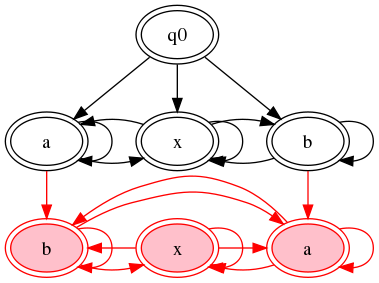The diagram makes obvious that L(B) is a proper subset of L(A): there are no blue states or arcs.

 By chromatic function I mean no more than that it is a function which maps elements of its domain into a set of colors.

 Brzozowski derivatives are defined by [Brzozowski 1964]; some readers may find the recapitulation in XML terms in [Sperberg-McQueen 2005] helpful, or at least easier to lay hands on; see also the literature cited there.

 All of the diagrams shown here were produced using an automatic graph-drawing tool (GraphViz), but almost all benefited from layout hints supplied by hand. Automatic calculation of useful layout hints would almost certainly help make diagrams for complex (i.e. realistic) content models easier to read.